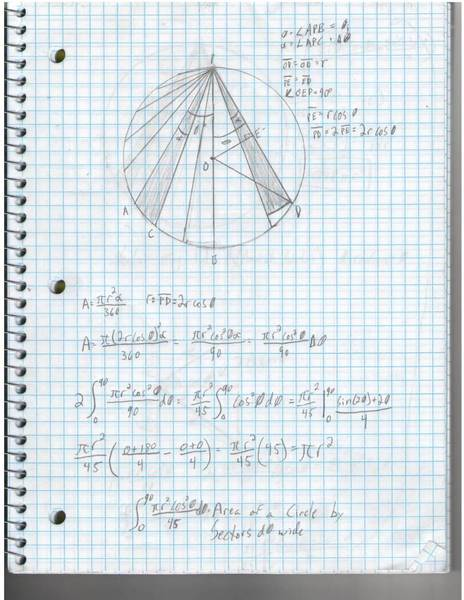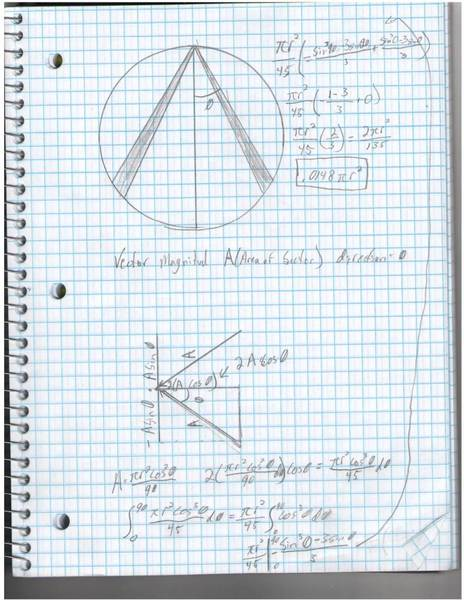# Area of a circle's perpendicular components

I asked a similar question about gravity, but I would just like to check the math of this first.In the first picture I have a circle of radius r, There is a point p on the circle, and I am first trying to show that the equation below gives the area of the circle:

$2\int^{90}_{0} \frac{\pi r^2cos^2\theta}{90}d\theta$

I got this from the area of a sector of a circle, but rather than from the center, go with the sector of a circle whose radius is a chord. It cuts some off, but as d$\theta$→0 so does the error.

If you take out the constants and solve, you have:

$\frac{\pi r^2}{45}\int^{90}_{0}\cos^2\theta d\theta = \frac{\pi r^2}{45} |^{90}_{0}\frac{sin(2\theta) + 2\theta}{4} = \frac{\pi r^2}{45}(\frac{180}{4}) = \pi r^2$

So that gives you the area of a circle figured up by little slices, the angle of each we know, it is $\theta$, or to be more correct, in each sector there is an angle $\theta^{\ast}_{\imath}$ that points to the center of the area, and as $d\theta$ approaches 0, $\theta^{\ast}_\imath$ approaches $\theta$

Alright, so if that is all good, then I introduced a vector, whose magnitude was the area of the sector defined above, and whose direction is $\theta$, relative to a line going through the point and the center, for each slice. I could figure out how much of the area is parallel to a line going from the point P to the center of the circle with:$2A\cos \theta$

Substituting in for A we get:
$\int^{90}_{0}\frac{\pi r^2\cos^3\theta}{45}d\theta$

As before pull out the constants and evaluate:

$\frac{\pi r^2}{45}\int^{90}_{0}cos^3\theta d\theta = \frac{\pi r^2}{45}|^{90}_{0}-\frac{\sin^3\theta -3\sin\theta}{3} = \frac{\pi r^2}{45}(\frac{2}{3}) = .0\overline{148}\pi r^2$

This this math correct? Is there only $1.\overline{481}\%$ of the circle "parallel to" a line going from the center to a point on the circumference?

*bump* Ah, I already feel this going unanswered...if someone could please check my math I would greatly appreciate it. And correction on the title, parallel is what I meant. Also, I dropped the $d\theta$ from the A= equation on the second page, but it should be there.